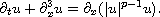Electron. J. Diff. Equ., Vol. 2015 (2015), No. 51, pp. 1-5.

### Nonexistence of soliton-like solutions for defocusing generalized KdV equations Soonsik Kwon, Shuanglin Shao

Abstract:
We consider the global dynamics of the defocusing generalized KdV equationWe use Tao's theorem  that the energy moves faster than the mass to prove a moment type dispersion estimate. As an application of the dispersion estimate, we show that there is no soliton-like solutions with a certain decaying assumption.

Submitted May 21, 2013. Published February 24, 2015.
Math Subject Classifications: 35Q53.
Key Words: Generalized KdV equation; soliton, scattering.

Show me the PDF file (181 KB), TEX file, and other files for this article.Soonsik Kwon Department of Mathematical Sciences Korea Advanced Institute of Science and Technology 291 Daehak-ro Yuseong-gu, Daejeon 305-701, Korea email: soonsikk@kaist.edu Shuanglin Shao Department of Mathematics, University of Kansas Lawrence, KS 66045, USA email: slshao@math.ku.edu